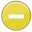#kilo 中文解釋 wordnet sense Collocation Usage Collins Definition
Noun
/ˈkēlō/,Font size:kilos, plural;
1. A kilogram

2. A kilometer

3. A code word representing the letter K, used in radio communication

1. kilogram: one thousand grams; the basic unit of mass adopted under the Systeme International d'Unites; "a kilogram is approximately 2.2 pounds"
2. Kilo- (symbol: k, lowercase) is a unit prefix in the metric system denoting one thousand. For example: * one kilogram is 1000 grams * one kilometre is 1000 metres * one kilojoule is 1000 joules * one kilobaud is a rate of transfer used primarily in telecommunications * one kilobyte is equal to ...
4. Short form of kilogram; The letter K in the ICAO spelling alphabet
5. A prefix used to indicate 1000 of the succeeding unit. (Kilo is also sometimes used as an abbreviation for kilogram.)
6. A numerical prefix denoting 1000.
7. A prefix indicating one (1) thousand.
8. A prefix meaning 1000. When used to measure computer data, it means 210 or 1024, which is the power of 2 closest to 1000.
9. A prefix that multiplies a basic unit by 1,000.
10. The lower case letter k indicates the resistance being measured is displayed in kilo-ohms (thousand (10^3)). The prefix kilo- is from the Greek word khilioi "thousand".
11. This is a unit of measure = 1,000.  So 1,000 bytes is a KiloByte.
12. 10^3.  One thousand.  See the Hacker's Dictionary for the full list of quantifiers.
13. A thousand. For instance, a kilowatt (kW) is 1,000 watts of power. A kilowatt hour (kWh) is the energy produced by a 1 kW generator in 1 hour.
14. A prefix meaning 1024 in computing terms. A kilobyte is therefore 1024 bytes. (Computing numbers are calculated in powers of 2, 2^10 in this case.)
15. A kilo generally means 1,000, but we say a kilo is 2^10 = 1,024.
16. a prefix used in the metric system of measurement to represent 1,000 (e.g., 1 kilogram (kg) = 1,000 grams, 1 kilojoule (KJ) = 1,000 joules).
17. 1 kilo = 2.228lbs, measurement often used in powder blends.
18. meaning 'thousand', as in kilogram or kilometer; see KLICK. Also, the word assigned to represent the letter "K" in the international phonetic alphabet; at various times in different spelling schemes, it has also been acrophonetically represented as King. See ALPHABET SOUP, PHONETIC ALPHABET. ...
19. Literally 1000. In binary terms though 1 Kilobyte (Kb) is 1024 bytes.
20. A metric unit representing 1000 (a thousand).
21. prefix denoting thousands or 1,000 or 10 to the 3rd power.
22. A multiplier indicating one thousand (1,000) of some unit. Abbreviated as k or K. When used to indicate a number of bytes of memory storage, the multiplier definition changes to 1,024. One kilobit, for example, equals 1,000 bits, whereas one kilobyte equals 1,024 bytes.
23. the metric prefix for 10^3, or times 1,000
24. Kilo is the greek prefix that means "thousand", as in kilobyte (KB), kilobit (Kb), kilowatt (KW), etc.   Since computers use the binary number system, a "kilo" in the computer world is actually 1024 (2^10).   See Mega.
25. (1) Greek word meaning 1000. (2) Prefix in the international system of measurements. (Example: kilohertz, kilowatts, etc.)